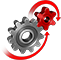# WolframSystemModeler

## Buck Converter

Using a linear DC‐to‐DC regulator is one of the simplest ways to reduce the voltage of a DC supply. However, as linear regulators work by converting excess power to heat, they are highly inefficient and inconvenient to use in many applications. A common alternative to reduce DC voltage is instead to use a buck converter. Buck converters can be found in a large number of applications, including the conversion of the main voltage in computers to a lower level that can be used by processors.

To run this example, you'll need

### The Model

A buck converter is a switched-mode power supply that uses two switches (usually a diode and a transistor), an inductor, and a capacitor to convert direct current voltage from a higher to a lower level. When the switch is turned off, the inductor current freewheels through the diode. When the switch is turned on, the inductor current increases and doesn’t pass through the diode. In this example, we study the difference in efficiency between a buck converter and a simple (linear) Zener regulator when converting a supply voltage (Vin) of 24 V to an output voltage (Vout) of 12 V.
A model of a buck converter and Zener regulator created in SystemModeler from standard components.

### Simulation Results

Both the buck converter and Zener regulator produce an output voltage of 12 V and a load current of 1 A. Note, however, the ripple effect caused by the buck converter. Output ripple is one of the main disadvantages of using a switched-mode power supply.Output voltage. The inset shows the output voltage ripple.